Warning: Illegal string offset 'html' in /home/hsn/public_html/forum/cache/skin_cache/cacheid_1/skin_topic.php on line 909

Maths Help? :( - HSN forum# Maths Help? :(

2 replies to this topic

### #1Gmac

• Gender:Male
• Gender:Male

Posted 07 December 2008 - 10:10 PM

1st post here so go easy on meI'm really stuck with 4 questions. Any help would be great thanks#1) Factorise fully: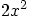+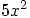- 4x - 3

#2) For what value of "a" does the equation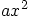+ 20x + 40 = 0

have equal roots?

#3) Show that the roots of the equation

(k-2)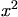- (3k-2)x + 2k = 0

are real?

#4)### #2Marcus

Site Swot

•• Members
•• 147 posts
• Location:Aberdeen
• Interests:Guitar - Passed my grade 6 :D :D<br />Write computer programs - won Heriot-Watt programming challenge 2006 and 2007 :D<br />
• Gender:Male

Posted 07 December 2008 - 11:19 PM

Question 1)

first I think you've made a typo I think it is supposed to be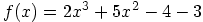, to find the first root is the most difficult, as it involves a bit of guesswork
you need to find a x value such that, f(x)=0, now x=1 works so (x-1) must be a factor then you need to use synthetic division
CODE
1 |  2  5  -4  -3
|     2   7   3
_________________
2  7   3   0

this tells us that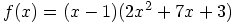now all we need to do is factorise that quadratic (if possible) now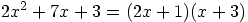soin its fully factorised form is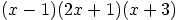Question 2)

now this question involves the discriminant, for equal roots b²-4ac=0
so a=a, b=20, c=40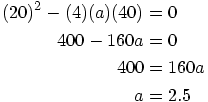Question 3)

this is another discriminant question, but for real roots b²-4ac >=0 or greater than or equal to zero
in this case a = (k-2), b= -(3k-2) c = 2k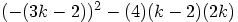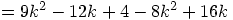now this is the challenge, to try and show that this quadratic is positive or zero for all values of k
Let's consider its graph
You can tell that if we put in a positive value this quadratic will be positive, so at least some of it is above the x-axis
if you factorise it you get (k+2)² which shows that its turning point is on the x-axis therefore it doesn't cross the x-axis.
Therefore, since part of the graph is above the x-axis, and it doesn't cross the x-axis (and the graph is continuous) therefore it is always above or equal to 0
Though this may be overkill compared to what you are expected to do

Question 4)
This is a nasty differentiation question for higher//now we need to turn that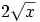into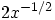and multiply the top line by it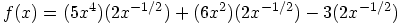//simplify using laws of indices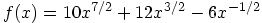then just differentiate like you would any other polynomial I'll let you do this yourself, if you struggle just post again and I'll write it out

Hope this helps, and I hope I haven't made any mistakes XD

=-=-=Marcus=-=-=

### #3Gmac

• Gender:Male
• Gender:Male

Posted 08 December 2008 - 05:59 PM

QUOTE (Marcus @ Dec 7 2008, 11:19 PM) <{POST_SNAPBACK}>
Question 1)

first I think you've made a typo I think it is supposed to be, to find the first root is the most difficult, as it involves a bit of guesswork
you need to find a x value such that, f(x)=0, now x=1 works so (x-1) must be a factor then you need to use synthetic division
CODE
1 |  2  5  -4  -3
|     2   7   3
_________________
2  7   3   0

this tells us thatnow all we need to do is factorise that quadratic (if possible) nowsoin its fully factorised form isQuestion 2)

now this question involves the discriminant, for equal roots b²-4ac=0
so a=a, b=20, c=40Question 3)

this is another discriminant question, but for real roots b²-4ac >=0 or greater than or equal to zero
in this case a = (k-2), b= -(3k-2) c = 2know this is the challenge, to try and show that this quadratic is positive or zero for all values of k
Let's consider its graph
You can tell that if we put in a positive value this quadratic will be positive, so at least some of it is above the x-axis
if you factorise it you get (k+2)² which shows that its turning point is on the x-axis therefore it doesn't cross the x-axis.
Therefore, since part of the graph is above the x-axis, and it doesn't cross the x-axis (and the graph is continuous) therefore it is always above or equal to 0
Though this may be overkill compared to what you are expected to do

Question 4)
This is a nasty differentiation question for higher//now we need to turn thatintoand multiply the top line by it//simplify using laws of indicesthen just differentiate like you would any other polynomial I'll let you do this yourself, if you struggle just post again and I'll write it out

Hope this helps, and I hope I haven't made any mistakes XD

Thanks Marcus

Really helped me understand

Owe you one mate!#### 1 user(s) are reading this topic

0 members, 1 guests, 0 anonymous users